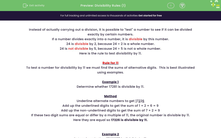# Understand the Rule for Divisibility by 11

In this worksheet, students will show their understanding of the rule for divisibility by 11.Key stage:  KS 3

Curriculum topic:   Number

Curriculum subtopic:   Use Concepts and Vocabulary for All Numbers

Popular topics:   Ratio worksheets

Difficulty level:#### Worksheet Overview

Instead of actually carrying out a division, it is possible to test a number to see if it can be divided exactly by certain numbers.

If a number divides exactly into a number, it is divisible by this number.

24 is divisible by 2, because 24 ÷ 2 is a whole number.

24 is not divisible by 5, because 24 ÷ 5 is not a whole number.

Here is the rule to test divisibility by 11.

Rule for 11

To test a number for divisibility by 11, we must find the sum of alternate digits.  This is best illustrated using examples.

Example 1

Determine whether 17,281 is divisible by 11.

Method

Underline alternate numbers to get 17226

Add up the underlined digits to get the sum of 1 + 2 + 6 = 9

Add up the non-underlined digits to get the sum of 7 + 2 = 9

If these two digit sums are equal or differ by a multiple of 11, the original number is divisible by 11.

Here they are equal, so 17,226 is divisible by 11.

Example 2

Determine whether 17,281,357 is divisible by 11.

Method

Underline alternate numbers to get 17281539

Add up the underlined digits to get the sum of 1 + 2 + 1 + 3 = 7

Add up the non-underlined digits to get the sum of 7 + 8 + 5 + 9 = 29

If these two digit sums are equal or differ by a multiple of 11, the original number is divisible by 11.

Here they differ by 29 - 7 = 22 so 17,281,539 is divisible by 11.

That's a pretty useful tip, isn't it?!Let's get to work!

### What is EdPlace?

We're your National Curriculum aligned online education content provider helping each child succeed in English, maths and science from year 1 to GCSE. With an EdPlace account you’ll be able to track and measure progress, helping each child achieve their best. We build confidence and attainment by personalising each child’s learning at a level that suits them.

Get started#### Popular Maths topics

••••